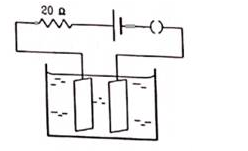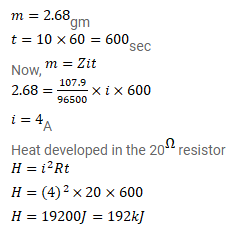# Figure shows an electrolyte of AgCl through which a current is passed.

Question:

Figure shows an electrolyte of $\mathrm{AgCl}$ through which a current is passed. It is observed that $2.68 \mathrm{~g}$ of silver is deposited in 10 minutes on the cathode. Find the heat developed in the $20^{\Omega}$ resistor during this period. Atomic weight of silver is $107.9$ $\mathrm{g} /$ mole.Solution: